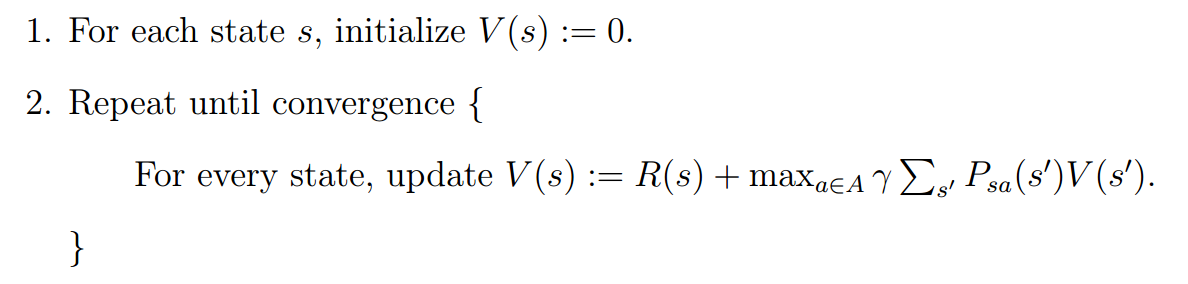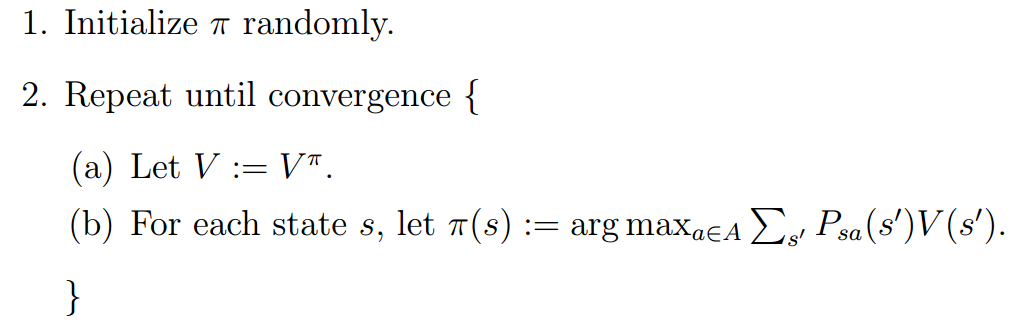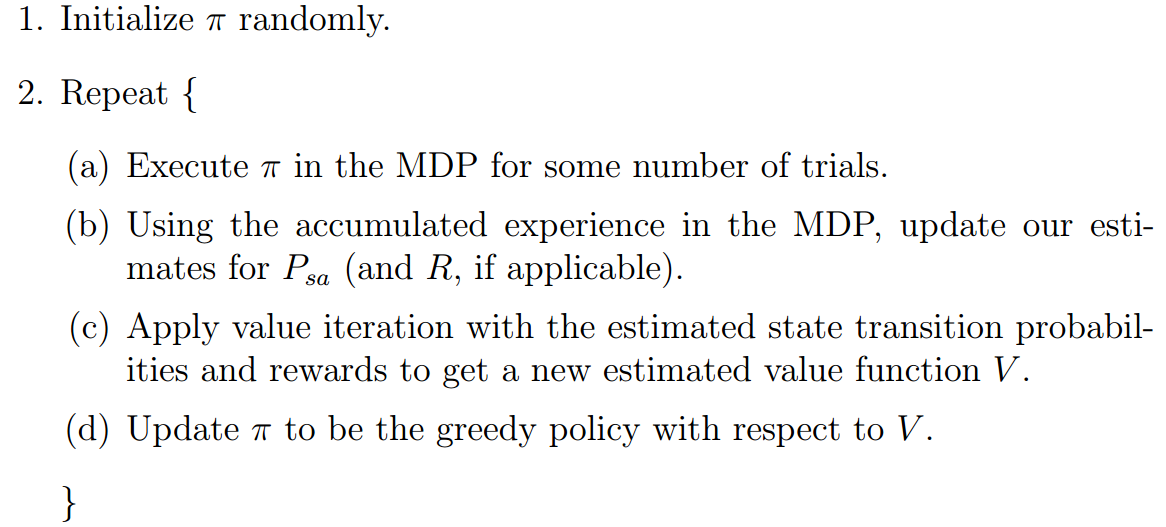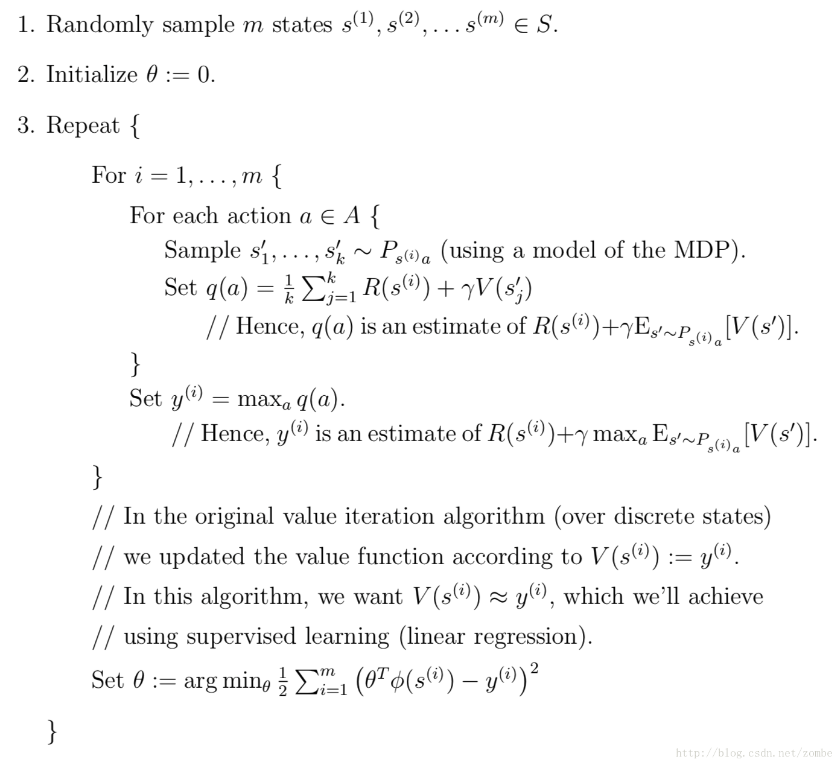# 一、离散状态的马尔科夫决策

### 2. Bellman方程

$V^{\pi} = R(s) + \gamma \sum_{s^{'}\epsilon S } P_{s\pi(s)}(s^{'})V^\pi (s^{'})$

### 3. 值迭代### 4. 政策迭代### 6. 转移概率和奖励因子的获取

if R is unknown, we can also pick our estimate of the expected immediate reward R(s) in state s to be the average reward observed in state s.

### 7. MDP求解全过程# 二、连续状态的马尔可夫决策

### 1、拟合值迭代算法### 2、近似政策迭代算法

posted @ 2018-11-19 20:32  天涯惟笑  阅读(331)  评论(0编辑  收藏  举报